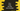# C++ program to find the nth even number## C++ program to print nth even number:

A number is called a even number if it is divisible by 2. The even numbers are 2, 4, 6, 8, 10, 12, 14… etc. For example, if the value of n is 4, it will print 8.

The easiest way to solve this problem by multiplying the value of n by 2. We can take the value of n as input from the user and print out the result.

### C++ program to find the nth even number:

Below is the complete C++ program:

``````#include <iostream>
using namespace std;

int main()
{
int n;

cout << "Enter the value of n : " << endl;
cin >> n;

cout << "nth Even number is : " << n * 2 << endl;
}``````

Here,

• n is an integer variable to store the value of n.
• Using cin, we are reading the user input value and storing it in n
• The last cout is used to print the nth even number, which is n * 2.

It will print output as like below:

``````Enter the value of n :
5
nth Even number is : 10``````

### C++ program to find the nth even number using a separate function:

We can also use a separate function to calculate the nth even number. For example:

``````#include <iostream>
using namespace std;

int getNthEven(int n)
{
return n * 2;
}

int main()
{
int n;

cout << "Enter the value of n : " << endl;
cin >> n;

cout << "nth Even number is : " << getNthEven(n) << endl;
}``````

Here,

• getNthEven function takes one integer value and returns the nth even number i.e. n * 2

It will print similar output.